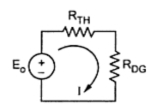### Ring Main Distributor With Interconnector

It has been mentioned that in ring main system, the cable is arranged in the loop or ring fashion. In most simple case, the ring distributor is fed at only one point.

But sometimes the ring main system is used to supply a large area and hence voltage drop across the various sections may become large in such case. Hence to compensate for such excessive voltage drops, the distant points of ring distributor are joined together by a conductor. This is called an interconnector. The Fig. 1 shows a ring main system with an interconnector.Fig. 1

The points D and G are joined by an interconnector.
Such a case is generally analysed using Thevenin's theorem.
Let us briefly revise the steps to use the Thevenin's theorem.
The steps to use Thevenin's theorem :
1. Remove an interconnector DG.
2. Find the voltage VDG without an interconnector, which is Thevenin's voltage denoted as Eo.
3. Determine the equivalent resistance as viewed through the terminals D and G, i.e. where an interconnector is to be connected. This is Thevenin's equivalent resistance denoted as RTH.
4. Knowing the resistance of an interconnector DG, the Thevenin;s equivalent can be drawn as shown in the fed Fig. 2.Fig. 2

5. The current I through an interconnector then can be obtained as,

Once this current is known, current in all the sections and the voltages at load points can be determined.

1.2.